Technical Article

# ADC INL Errors—Best Fit Line, Total Unadjusted Error, Absolute and Relative Accuracy

December 30, 2022 by Dr. Steve Arar

Integral nonlinearity (INL) an important specification that allows us to characterize the static linearity performance of an A/D (analog-to-digital) converter. The INL error quantifies the deviation of the transition points of the actual transfer function from the ideal values, which are obtained from a reference straight line. However, different INL definitions use different reference lines.

Previously, we looked at some of these definitions, like the endpoint-based definitions. As a refresher, the reference line for the most common INL definition is the line that goes through the first and last code transitions (the line going through points A and B in Figure 1).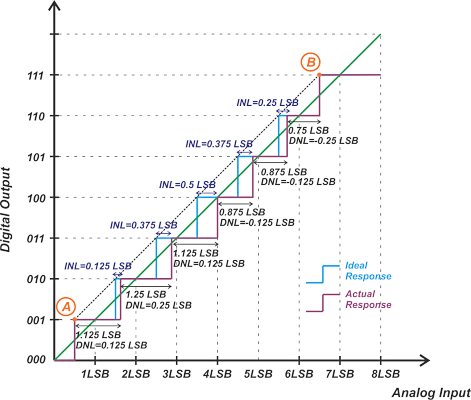##### Figure 1. Example reference line INL definition.

The above INL definition is categorized as an endpoint method because it only uses the first and last codes for deriving the reference line. In this article, we'll cover another method for defining the INL error, the best fit line method. In this case, a straight line fit through all codes is used as the reference line.

### Transition Best Fit INL—Endpoint Method vs. Best Fit Method

Regardless of using the endpoint or best fit method, the static transfer characteristic of an ADC can be defined in terms of the code centers or the transition points. The reference line for the transition-based best fit INL definition is the straight line that best represents all transition points of the characteristic curve. Let’s consider the non-ideal response in Figure 1, which is reproduced in Figure 2.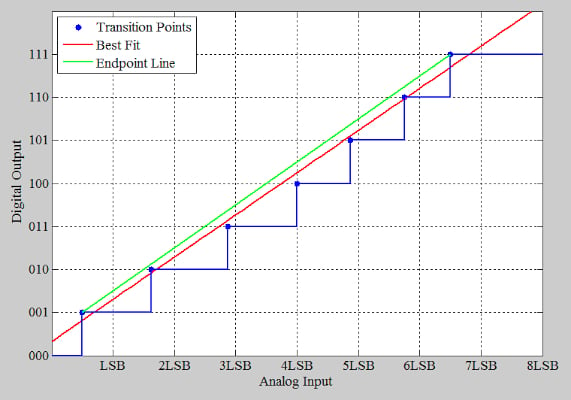##### Figure 2. Example of a non-ideal response.

In Figure 2, the dots represent the transition points of the characteristic curve, and the green line is the endpoint line that goes through the first and last transition points. Obviously, a straight line cannot go through all these transition points. However, we can find the straight line that “best fits” our data points (the red line in the figure). The least-squares method is used to find the line of best fit.

The least-squares method is a statistical procedure that fits a line to the data by minimizing the sum of the offsets (or “residuals”) of the points from the straight line. The calculations involved in the least-squares method are tedious, and oftentimes, a spreadsheet or a computer program is used to do these calculations.

As an example, illustrated in Figure 2, calculating the deviations of the transition points from the best fit line leads to the following INL plot.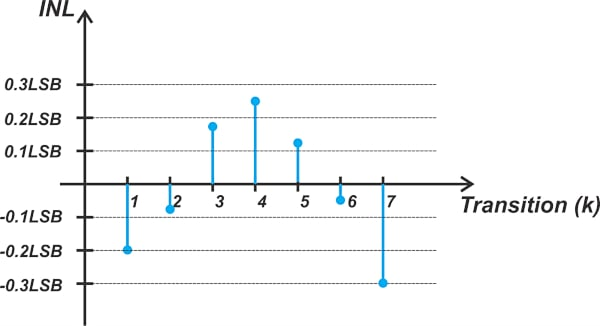##### Figure 3. Example INL plot from calculating the transition point deviations from the best fit line.

For this hypothetical ADC, applying the endpoint method leads to a maximum INL error of +0.5 least significant bits (LSBs), as shown in Figure 1. With the best fit method, however, the absolute value of the INL error is less than 0.3 LSBs, which is almost half that of the endpoint method. Figure 4 should help you better visualize how the best fit method makes a given characteristic curve seem to be more linear.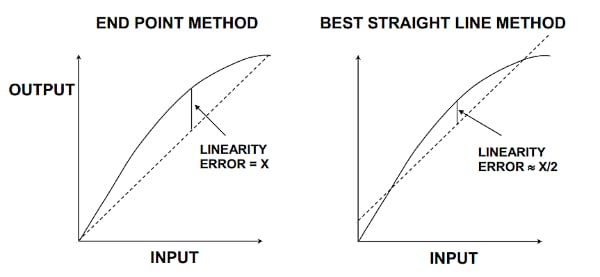##### Figure 4. Examples showing the endpoint method (left) and best straight-line method (right). Image used courtesy of Analog Devices [PDF]

In Figure 4, the solid curve represents the nonlinear ADC response. As you can see, the best fit method inherently chooses a reference line that minimizes the maximum INL error and tends to hide the details of linearity performance. As a result, the best fit method doesn’t seem to be really useful when analyzing the error budget of a measurement system. This is because, for error budget analysis, we need to calculate the deviations from the ideal transfer characteristic, not from some arbitrary "best fit."

While the endpoint method better suits measurement and control applications, the best fit method could provide a better prediction of distortion in AC applications. However, even for AC applications, we normally prefer to use specifications such as harmonic distortion and spurious-free dynamic range (SFDR) because these metrics can characterize the dynamic linearity of the system.

Therefore, it is rarely necessary to use the best fit INL specification. You need to be familiar with it as you might occasionally run into devices that are characterized using a best fit method.

### Code Center Best Fit INL

For the sake of completeness, the code center-based INL definition is also shown below.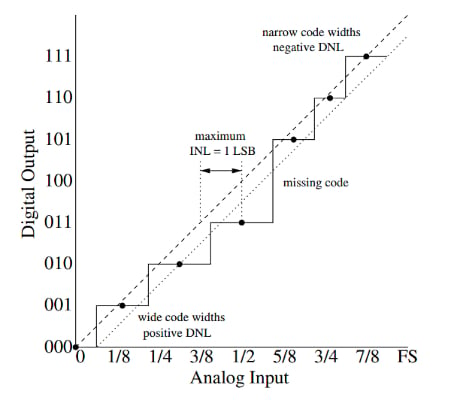##### Figure 5. Example of a code center-based INL. Image used courtesy of K. Lundberg [PDF]

In this figure, the dashed line is the linear model of the ADC, and the dotted line is the line that fits the actual code centers. The INL is defined as the distance of the code centers from the reference line. For the endpoint method, the reference line is the dashed line. For the best fit method, however, the reference line is the dotted line. Again, the best fit method inherently hides the system's nonlinearity and can produce a much smaller INL error than the endpoint method.

Now that we’re familiar with the INL specification, we can discuss how the accuracy of an ADC is defined. In the context of ADCs, accuracy is not actually a well-defined parameter, and there are some inconsistencies that we’ll discuss shortly. “Absolute accuracy,” “relative accuracy,” and “total unadjusted error” are three common methods of describing the transfer function accuracy in ADCs.

This TI document defines the “absolute accuracy” of an ADC at a given code as the maximum difference between the analog input that produces that particular code in the actual response from the ideal code center. This is best illustrated graphically in Figure 6 (which is reproduced from the article I mentioned above).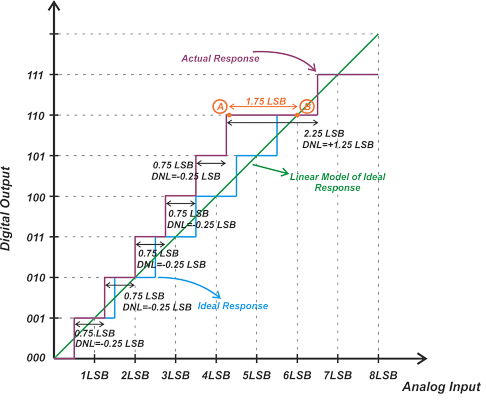##### Figure 6. Plot showing the absolute accuracy of an ADC.

In this example, an analog input of 4.25 LSBs (which corresponds to point A) is the smallest value that produces code 110. The analog equivalent of code 110 is 6 LSBs. This means that, for an input of 4.25 LSBs, the ADC outputs 6 LSBs, leading to an absolute accuracy error of 1.75 LSBs. As you can see, this error can be found by calculating the difference between point A and the ideal code center (point B). Figure 7 shows another example of applying this accuracy definition.##### Figure 7. An additional example of applying absolute accuracy. Image used courtesy of TI [PDF]

With the above “absolute accuracy” definition, four different error sources, namely offset error, gain error, INL error, and quantization error, are included. However, keep in mind that sometimes the absolute accuracy definition provided in certain references does not include the quantization error. For example, the popular textbook “Analog Integrated Circuit Design” defines absolute accuracy as the difference between the expected and actual transfer responses. The book further elaborates that absolute accuracy includes offset, gain, and linearity errors.

Additionally, the book “The Data Conversion Handbook” also doesn’t include the quantization error and defines absolute accuracy as the difference between the actual and ideal code centers. For example, if the values in the range 5V ±1.2 mV produce a given code in the ideal response and the actual converter produces that code for inputs from 4.997 V to 4.999 V, then the absolute error is obtained as:

$Absolute \text{ } Error=\frac{1}{2}\big(4.997+4.999)-5=-2 \text{ }mV$

This definition is similar to the code center-based INL definition; however, the difference is that it calculates the error without nullifying the offset and gain errors.

“Relative accuracy” is another term for the maximum INL error. Therefore, the relative accuracy expresses the ADC accuracy after its offset and gain errors are calibrated out.

TUE is another way to express the total accuracy of an ADC. The TUE is also the combined effect of offset, gain, and INL errors. However, it is not calculated as a summation of these errors. The maximum TUE is normally calculated as the root-sum-square (RSS) of the maximum values of the offset, gain, and INL errors:

$TUE=\sqrt{(Offset \text{ } Error)^2+(Gain \text{ }Error)^2+ INL^2}$

The TUE can also be used to express the accuracy of the whole acquisition system. In this case, the offset and gain errors from other blocks, such as the input driver, voltage reference, etc., are also included.

### Key Takeaways on ADC INL Errors

• The INL error quantifies the deviation of the actual transfer function from the ideal response. Different INL definitions use different reference lines to define the ideal response. INL definitions can be categorized as endpoint and best fit methods.
• While the endpoint method uses the first and last codes for deriving the reference line, the best fit method uses a line of best fit as its reference.
• Regardless of using the endpoint or best fit method, the static transfer characteristic of an ADC can be defined in terms of the code centers or the transition points (for INL calculations).
• The accuracy of an ADC is normally expressed using three specifications: absolute accuracy, relative accuracy, and TUE.

Featured image used courtesy of Adobe Stock